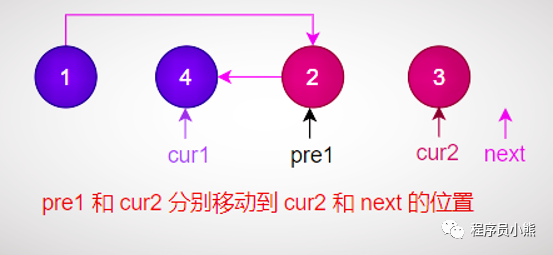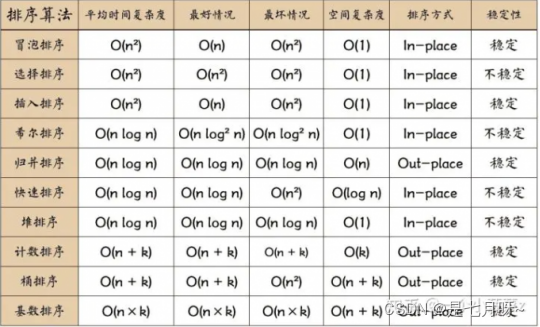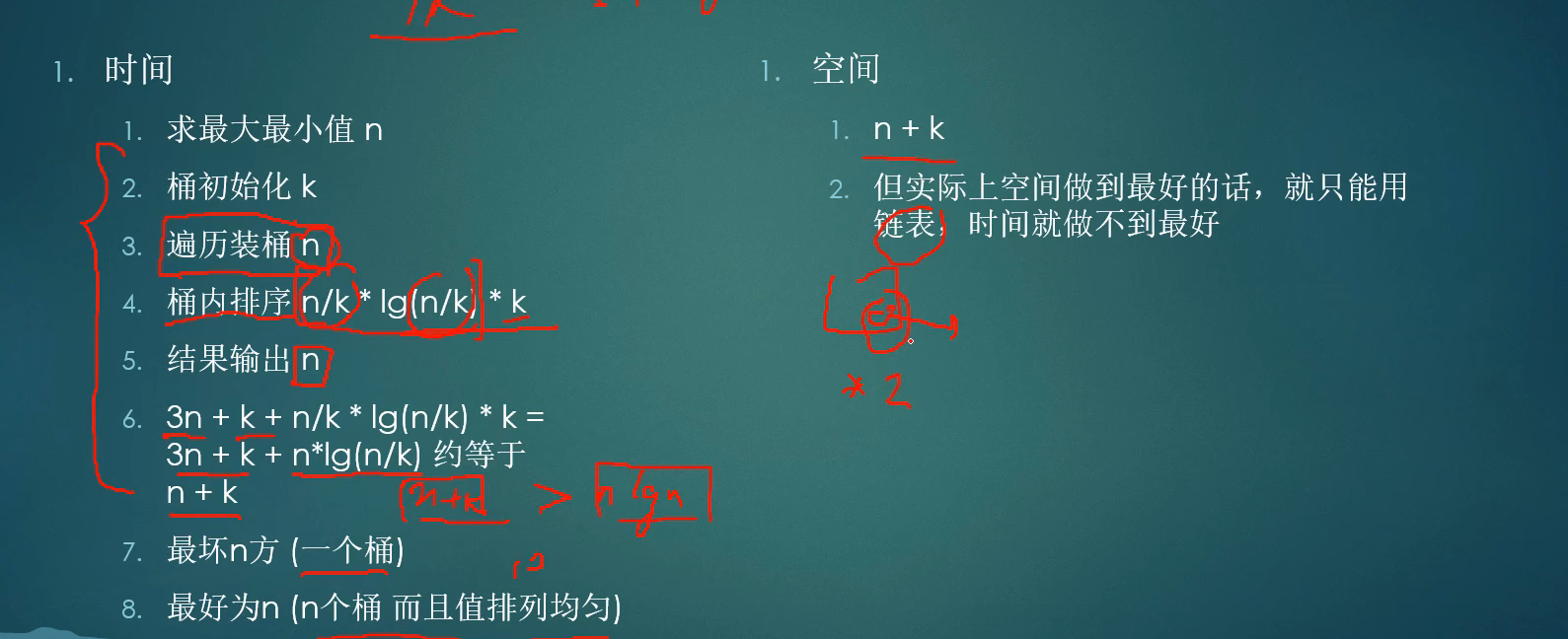# 数据结构和算法常见面试问题总结，含答案

+关注继续查看

### 5. 链表升序合并### 12. 二叉树的最近公共祖先

TreeNode* lowestCommonAncestor(TreeNode* root, TreeNode* p, TreeNode* q) {
if(root==p||root==q||!root)return root;

TreeNode* left=lowestCommonAncestor(root->left,  p, q);
TreeNode* right=lowestCommonAncestor(root->right,  p, q);

if(!left&&!right)return NULL;
else if(left&&!right)return left;
else if(right&&!left)return right;

return root;
}



### 16. 图的应用

#### 最短路径：

Dijkstra时间复杂度O(n2) 适用于 权值为非负 的图的单源最短路径，用斐波那契堆的复杂度O(E+VlgV)，空间

Floyd时间复杂度O(n3)，空间复杂度O(n)

#### prim算法

Prim时间复杂度为O(n2)，适用于稠密图

prim算法的思想是，从一个起点开始，通过不断的纳入与当前生成树距离最短的且不在生成树中的点来构造最小生成树，当所有点都纳入到生成树以后，就得到了最小生成树。使用邻接表来存储图，能使得所需的空间最小。通过一个数组来存放各个点到当前生成树的最短距离，可以避免重复比较，从而提升效率。但我们所要获取的是该数组里的距离最小的那个点。如果没有进行堆优化，那从这个数组里找出最小距离的点的时间复杂度是O（n）,通过堆优化，我们获取最小值的时间复杂度则为O（log2n）,在图的点数目很大时，能有效的提升效率。

Kruskal：并查集的贪心算法，时间复杂度为O(ElogE)

### 17. 查找算法

#### B树和B+树

B树特性：

B树好处：

B树的每一个结点都包含key(索引值) 和 value(对应数据)，因此方位离根结点近的元素会更快速。（相对于B+树）

B树的不足：

#### KMP

next数组标记的是，匹配字符串当前索引及其之前的子串最大长度相同前缀和后缀的数量

### 18. 排序算法1，利用分治思想

2，具体实现都用递归

#### 桶排序### 21. 求数组最大子序列和

①如果前面的累加值为负数或者等于0，那对累加值清0重新累加，把当前的第i个数的值赋给累加值。

②如果前面的累加值为整数，那么继续累加，即之前的累加值加上当前第i个数的值作为新的累加值。

### 22. 快排算法

T[n] = 2T[n/2] + f(n)；T[n/2]为平分后的子数组的时间复杂度，f[n] 为平分这个数组时所花的时间

public class QuickSort {
public static void sort(int a[], int low, int hight) {
int i, j, index;
if (low > hight) {
return;
}
i = low;
j = hight;
index = a[i]; // 用子表的第一个记录做基准
while (i < j) { // 从表的两端交替向中间扫描
while (i < j && a[j] >= index)
j--;
if (i < j)
a[i++] = a[j];// 用比基准小的记录替换低位记录
while (i < j && a[i] < index)
i++;
if (i < j) // 用比基准大的记录替换高位记录
a[j--] = a[i];
}/
a[i] = index;// 将基准数值替换回 a[i]
sort(a, low, i - 1); // 对低子表进行递归排序
sort(a, i + 1, hight); // 对高子表进行递归排序

}

public static void quickSort(int a[]) {
sort(a, 0, a.length - 1);
}

public static void main(String[] args) {

int a[] = { 49, 38, 65, 97, 76, 13, 27, 49 };
quickSort(a);
System.out.println(Arrays.toString(a));
}
}


### 23. 归并排序

import java.util.Arrays;

public class MergeSort {
public static void main(String[] args) {
int[] arr = {11,44,23,67,88,65,34,48,9,12};
int[] tmp = new int[arr.length];    //新建一个临时数组存放
mergeSort(arr,0,arr.length-1,tmp);
System.out.println(Arrays.toString(arr));
}
public static void merge(int[] arr,int low,int mid,int high,int[] temp){
int i=0;
int j=low,k=mid+1;
while(j<=mid&&k<=high){
if(arr[j]<=arr[k]){
temp[i++]=arr[j++];
}else{
temp[i++]=arr[k++];
}
}
while(j<=mid){
temp[i++]=arr[j++];
}
while(k<=high){
temp[i++]=arr[k++];
}
for(int t=0;t<i;t++){
arr[low+t]=temp[t];
}
}
public static void mergeSort(int[] arr,int low,int high,int[] temp){
if(low<high){
int mid=(low+high)/2;
mergeSort(arr, low, mid, temp);
mergeSort(arr, mid+1, high, temp);
merge(arr,low,mid,high,temp);
}
}

}


### 25. 循环引用怎么解决

「若 A 强引用了 B，那 B 引用 A 时就需使用弱引用，当判断是否为无用对象时仅考虑强引用计数是否为 0，不关心弱引用计数的数量」

### 26. 什么是拓扑排序|
2天前
|

【数据结构】算法的时间复杂度和空间复杂度
【数据结构】算法的时间复杂度和空间复杂度
20 0
|
5天前
|

C语言数据结构2 1.2 算法
C语言数据结构2 1.2 算法
32 0
|
6天前
|

C++算法：全 O(1) 的数据结构
C++算法：全 O(1) 的数据结构
13 0
|
8天前
|

【Python深入学习】- 书籍推荐|数据结构和算法介绍|内建集合数据类型
【Python深入学习】- 书籍推荐|数据结构和算法介绍|内建集合数据类型
31 1
|
9天前
|

【算法与数据结构】二叉树的三种遍历代码实现（上）—— 用递归序知识点讲解
【算法与数据结构】二叉树的三种遍历代码实现（上）—— 用递归序知识点讲解
9 0
|
9天前
|

【算法与数据结构】归并排序的代码实现（详细图解）以及master公式的讲解
【算法与数据结构】归并排序的代码实现（详细图解）以及master公式的讲解
19 0
|
9天前
|

【算法与数据结构】二叉树的三种遍历代码实现（下）—— 非递归方式实现（大量图解）
【算法与数据结构】二叉树的三种遍历代码实现（下）—— 非递归方式实现（大量图解）
7 0
|
16天前
|

13 0
|
16天前
|

16 1
|
16天前
|

20 1